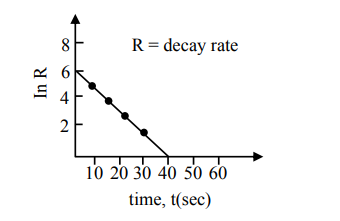# For a certain radioactive process the graphQuestion:

For a certain radioactive process the graph between In $\mathrm{R}$ and $\mathrm{t}(\mathrm{sec})$ is obtained as shown in the figure. Then the value of half life for the unknown radioactive material is approximately:1. $9.15 \mathrm{sec}$

2. $6.93 \mathrm{sec}$

3. $2.62 \mathrm{sec}$

4. $4.62 \mathrm{sec}$

Correct Option: , 4

Solution:

$\mathrm{R}=\mathrm{R}_{0} \mathrm{e}^{-\lambda \mathrm{t}}$

$\ell \mathrm{nR}=\ell \mathrm{nR}_{0}-\lambda \mathrm{t}$

$-\lambda$ is slope of straight line

$\lambda=\frac{3}{20}$

$\mathrm{t}_{1 / 2}=\frac{\ell \ln 2}{\lambda}=4.62$